Example Questions

Example Question #7 : How To Find The Equation Of A Parallel Line

Which of these formulas could be a formula for a line perpendicular to the line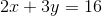?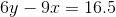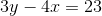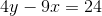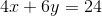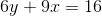Explanation:

This is a two-step problem. First, the slope of the original line needs to be found. The slope will be represented by "" when the line is in-intercept form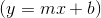.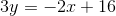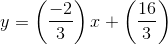So the slope of the original line is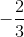. A line with perpendicular slope will have a slope that is the inverse reciprocal of the original. So in this case, the slope would be. The second step is finding which line will give you that slope. For the correct answer, we find the following: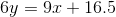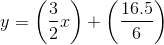So, the slope is, and this line is perpendicular to the original.

Example Question #11 : Parallel Lines

Which of the following equations is parallel to: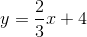and goes through the point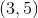?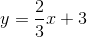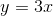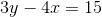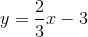Explanation:

Step 1: We need to define what a parallel line is. A parallel line has the same slope as the line given in the problem. Parallel lines never intersect, which tells us that the y-intercepts of the two equations are different.

Step 2: We need to identify the slope of the line given to us. The slope is always located in front of the.

The slope in the equation is.

Step 3: If we said that a parallel line has the same slope as the given line in the equation, the slope of the parallel equation is also.

Step 4. We need to write the equation of the parallel line in slope-intercept form:. We need to write b for the intercept because it has changed.

The equation is: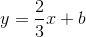Step 5: We will use the pointwhereand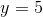. We need to substitute these values of x and y into the equation in step 4 and find the value of b.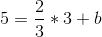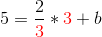The numbers in red will cancel out when I multiply.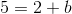To find b, subtract 2 to the other side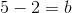Step 6: We put all of the parts together and make the final equation of the parallel line:

The final equation is:Example Question #8 : Parallel Lines

Consider line c to be y= -4x - 7. Which is the reflection of line c across the x-axis?

y = 4x – 7

y = (1/4)x + 7

y = 4x + 7

y = –4x + 7

y = 4x + 7

Explanation:

A line reflected across the x-axis will have the negative value of the slope and intercept. This leaves y= 4x + 7.

Example Question #9 : Parallel Lines

If line J passes through the points (1, 3) and (2, 4) and line K passes through (0, x) and (10, 3), what would be the value of x in order for lines J and K to be parallel?

1

–7

13

7

–13

–7

Explanation:

Find the slope of line J, (4 – 3)/(2 – 1) = 1

Now use this slope in for the equation of line K of the form y = mx + b for the other point (10, 3)

3 = 10 + b → b = –7

So for the point (0, X) → X = 0 – 7,

so x = –7 when these two lines are parallel.

Example Question #10 : Parallel Lines

Lineis represented by the equation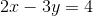.

If linepasses through the points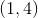and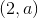, and ifis parallel to, then what is the value of?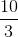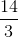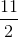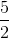Explanation:

We are told that lines l and m are parallel. This means that the slope of line m must be the same as the slope of l. Line l is written in the standard form of Ax + By = C, so its slope is equal to –A/B, or –2/–3, which equals 2/3. Therefore, the slope of line m must also be 2/3.

We are told that line m passes through the points (1, 4) and (2, a). The slope between these two points must equal 2/3. We can use the formula for the slope between two points and then set this equal to 2/3.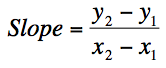slope = (a – 4)/(2 – 1) = a – 4 = 2/3

a – 4 = 2/3

Multiply both sides by 3:

3(a – 4) = 2

3a – 12 = 2

3a = 14

a = 14/3

Example Question #14 : Coordinate Geometry

What is the slope of a line parallel to the line: -15x + 5y = 30 ?

30

3

1/3

-15

3

Explanation:

First, put the equation in slope-intercept form: y = 3x + 6. From there we can see the slope of this line is 3 and since the slope of any line parallel to another line is the same, the slope will also be 3.

Example Question #15 : Coordinate Geometry

What is the slope of any line parallel to –6x + 5y = 12?

6

6/5

5/6

12/5

12

6/5

Explanation:

This problem requires an understanding of the makeup of an equation of a line.  This problem gives an equation of a line in y = mx + b form, but we will need to algebraically manipulate the equation to determine its slope.  Once we have determined the slope of the line given we can determine the slope of any line parallel to it, becasue parallel lines have identical slopes.  By dividing both sides of the equation by 5, we are able to obtain an equation for this line that is in a more recognizable y = mx + b form. The equation of the line then becomes y = 6/5x + 12/5, we can see that the slope of this line is 6/5.

Example Question #16 : Coordinate Geometry

What is the slope of a line that is parallel to the line 11x + 4y - 2 = 9 – 4x  ?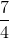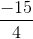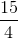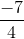Explanation:

We rearrange the line to express it in slope intercept form.

Any line parallel to this original line will have the same slope.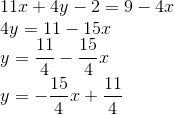Example Question #17 : Coordinate Geometry

In the standard (x, y) coordinate plane, what is the slope of a line parallel to the line with equation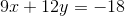?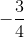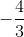Explanation:

Parallel lines will have equal slopes. To solve, we simply need to rearrange the given equation into slope-intercept form to find its slope.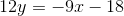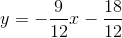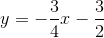The slope of the given line is. Any lines that run parallel to the given line will also have a slope of.

Example Question #18 : Coordinate Geometry

What is the slope of a line that is parallel to the line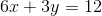?Explanation:

Parallel lines have the same slope. The question requires you to find the slope of the given function. The best way to do this is to put the equation in slope-intercept form (y = mx + b) by solving for y.

First subtract 6x on both sides to get 3y = –6x + 12.

Then divide each term by 3 to get y = –2x + 4.

In the form y = mx + b, m represents the slope. So the coefficient of the x term is the slope, and –2 is the correct answer.